Volume 1, Issue 1, July 2015, Pages: 6-9

Testing the FLUKA Monte Carlo Program Performance Using the Experimental Alpha Energy Spectrum

Elif Ebru Ermis*, Cuneyt Celiktas

Ege University, Faculty of Science, Physics Department, Bornova, Izmir/Turkey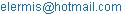(E. E. Ermis)

Elif Ebru Ermis, Cuneyt Celiktas. Testing the FLUKA Monte Carlo Program Performance Using the Experimental Alpha Energy Spectrum. Radiation Science and Technology. Vol. 1, No. 1, 2015, pp. 6-9.doi: 10.11648/j.rst.20150101.12

Abstract: The alpha energy spectra of 210Po were achieved through experimental and theoretical methods. The spectrometer which was consisted of silicon semiconductor surface barrier detector (SBD) was used to obtain experimental energy spectrum of the isotope. The theoretical alpha energy spectrum was also achieved through FLUKA Monte Carlo method to analyze and reveal its performance on alpha spectrum. Experimental and theoretical energy resolution values from the obtained spectra were determined and compared to each other. It was shown that, as a result of the comparison, experimental and theoretical energy spectra were comparable to each other according to spectral shape and the energy resolution. In conclusion, FLUKA MC program was quite successful in the determination of alpha energy spectrum of a radioactive source with satisfying energy resolution.

Keywords: 210Po, FLUKA, Silicon Surface Barrier Detector, Alpha Particles

Contents

1. Introduction

The most widely used silicon detectors for charged particle measurements are the surface barrier type. These detectors rely on the junction formed between a semiconductor and certain materials, usually n type silicon with gold or p-type silicon with aluminum (Leo, 1987).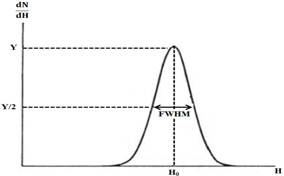Figure 1. Definition of detector resolution (De Lima, 2011).

A particle energy spectrum is a function giving the distribution of particles in terms of their energies (Tsoulfanidis, 1995). The detection performance of a detector is given in terms of energy resolution, it depends on the full width at half maximum (FWHM) of a peak in the energy spectrum. As clear from Fig. 1, energy resolution of the detector is conventionally defined as the FWHM divided by the location of the peak centroid H0 (Eq. 1). The energy resolution R is thus a dimensionless fraction conventionally expressed as a percentage (Knoll, 2000).

Resolution (R) = FWHM/H0                           (1)

It is clear that the smaller value for the energy resolution, the better the detector, as it will be able to distinguish between two close radiations whose energies lie close to each other (Knoll, 2000).

The interactions of radiations with matter can be simulated by Monte Carlo (MC) methods. MC simulation methods need some input data; for example, details of geometry of radiation source, target and medium, type of radiation, energy, and direction of radiation flight (De Lima, 2011).

ROOT works on C++. It is an object-oriented framework aimed at solving the data analysis challenges of high-energy physics. It is also used for advanced data analysis such as MC simulations, its user guide includes further information (ROOT, 2009).

Rahab et al. described a method using a silicon surface barrier detector which can be constructed with p- and n-type high purity silicon and Al as a rectifying contact (Rahab et al., 2001). Ullom et al. achieved the 210Po energy spectrum using microcalorimeter (Ullom et al., 2006). 210Po distribution in a lead disk was obtained by means of semiconductor detector by Misiaszek et al. (Misiaszek et al., 2013). GEANT4 MC method was also used to simulate geometry and spectrometer by Misiaszek et al., also, Shi et al. determined the gamma-ray response functions of a NaI(Tl) detector using MC simulations (Shi et al., 2002).

However, according to Authors’ literature survey, no study was available about the simulation of alpha particles energy spectrum using FLUKA MC method and the comparison with the experimental result. Therefore, additionally, it is of some importance to test the FLUKA MC program performance for alpha energy spectrum and its characteristics.

In the presented study, the comparison of theoretical and experimental energy spectra were also carried out to test the FLUKA performance on alpha particles energy spectrum.

2. Materials and Methods

FLUKA MC program (Ferrari et al., 2005; Battistoni et al., 2007) and the spectrometer which was consisted of a silicon semiconductor surface barrier detector were used to obtain the theoretical and experimental energy spectra of 210Po in this study.

5.40 MeV-energy alpha particles are emitted by the 210Po radioactive source. Its half-life is 138.376 days, and the activity of the used one was 0.1 µCi.

The experimental energy spectrum of 210Po was achieved through the spectrometer consisted of a silicon semiconductor surface barrier detector (Ortec, model no: CA-18-50-100). Its active area and thickness are 50 mm2 and 100 µm, respectively. A schematic diagram of a silicon semiconductor surface barrier detector is shown in Fig. 2.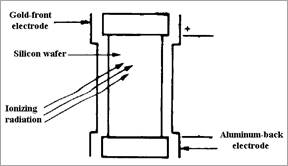Figure 2. A schematic representation of typical surface barrier detector (Tsoulfanidis, 1995).

Data acquisition time was 900s in the experiment. In order to stabilize the detector signal, the experimental spectrum was achieved at a constant room temperature (200C). Since alpha particles have small range in the air, used detector was placed into a vacuum chamber (about 500 µm vacuum level).

First, the detector output was sent to a preamplifier (PA, Ortec 142), second, the energy output of the PA was connected to a delay line amplifier (DLA, Ortec 460), then, its bipolar output was sent to a delay amplifier (DA, Ortec 427A), and finally, the output of the DA was connected to a multichannel analyzer (MCA, Ortec Trump 8K) to acquire the energy spectrum of the source (Fig. 3).Figure 3. Block diagram of the used spectrometer.

(HV: High voltage supplier, DT: Detector, PA: Preamplifier, DLA: Delay line amplifier, DA: Delay amplifier, MCA: Multichannel analyzer)

The output signal shape of the DLA is shown in Fig. 4.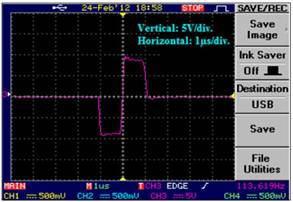Figure 4. Output signal shape of DLA.

In this work, the energy spectrum of the used radioactive source was also achieved through a theoretical method, where FLUKA MC program was run to obtain theoretical energy spectrum of the used source. Ubuntu (ver. 11.04) operating system was used to install FLUKA (ver. 2011.2b). In the program, geometrical values of the detector (diameter and thickness) were implemented so as to be the same as the experimental set-up in order to compare the theoretical spectrum to the experimental spectrum. Schematic view of the used detector formed in FLUKA is shown in Fig. 5.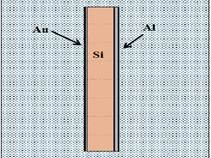Figure 5. A schematic of the detector created in FLUKA.

3. Results and Discussion

A rectangular bipolar signal shape (Fig. 4) was obtained from the spectrometer and used for the energy spectrum. Experimental energy spectrum which was achieved by the silicon semiconductor surface barrier detector of the used radioactive source is shown in Fig. 6. The number of channels and the counts per channel were corresponded to horizontal and vertical axes, respectively. Experimental energy resolution of the detector was calculated as 1.66%.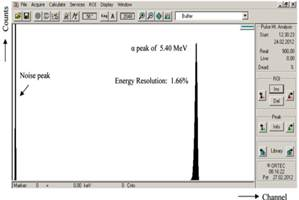Figure 6. Experimental energy spectrum of 210Po.

The theoretical energy spectrum of the used radioisotope was acquired over 1,000 particles by means of FLUKA MC program, as shown in Fig. 7, the theoretical alpha energy spectrum is represented. The energy resolution was calculated as 1.39% from this spectrum.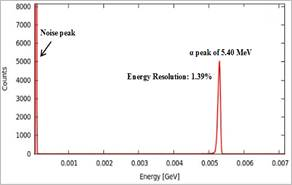Figure 7. Theoretical alpha energy spectrum from FLUKA program (GeV is the standard energy unit).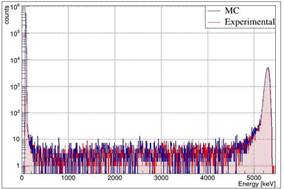Figure 8. Comparison of the obtained experimental energy spectrum with that of FLUKA via ROOT program.

In addition, the theoretical alpha energy spectrum was compared to the experimental one using ROOT (ver. 5.34/18) program. In order to check accuracy of the theoretical result, a comparison between the theoretical and the experimental results is given in Fig. 8.

4. Conclusions

The experimental energy resolution value of the spectrometer consisted of a silicon semiconductor surface barrier detector was found as 1.66% (Fig. 6). The energy spectrum of the used radioisotope was also obtained by FLUKA MC program where the theoretical energy resolution value was calculated as 1.39% (Fig. 7). In the MC program, some other effects such as background and electronic noise which was produced by the used electronic modules in the spectrometer could not be considered. Therefore, the theoretical energy resolution value was normally lower than the experimental one.

The comparison was made between the experimental and the theoretical energy spectra in this study. It was realized that experimental and theoretical spectra were in good accordance with each other from the point of view of energy spectrum. It is finally concluded that the alpha energy spectrum was calculated numerically using FLUKA MC program.

Acknowledgement

This work was supported by TUBITAK, the Scientific and Technical Research Council of TURKEY under project No. 197T087, Scientific Research Foundation of Ege University under project No. 11 FEN 085, Center of Science and Technology (EBILTEM) of Ege University under project No. 99 BIL 001 and 12 BIL 004.

References

1. Battistoni, G., Cerutti, F., Fasso, A., et al., 2007. ‘‘The FLUKA Code: Description and Benchmarking’’. AIP Conference Proceeding, 896 (31), 31-49.
2. De Lima, J.J.P., 2011. ‘‘Nuclear Medicine Physics’’. Taylor&Francis, USA.
3. Ferrari,A.,Sala, P.R.,Fasso,A.,etal.,2005. ‘‘Fluka:AMulti-particleTransportCode’’. CERN, INFN/TC_05/11, SLAC-R-773.
4. Knoll, G.F., 2000. ‘‘Radiation Detection and Measurement’’. John Wiley & Sons. Inc, New York.
5. Leo, R.W., 1987. ‘‘Techniques for Nuclear and Particle Physics Experiments’’. Springer–Verlag Berlin Heidelberg, Germany.
6. Misiaszek, M., Pelczar, K., Wójcik, M., et al., 2013. ‘‘Optimization of Low-background Alpha Spectrometers for Analysis of Thick Samples’’. Applied and Radiation and Isotopes. 81, 146-150.
7. Rahab, H., Keffous, A., Menari, H., et al., 2001. ‘‘Surface Barrier Detectors Using Aluminum on n- and p-type Silicon for α-spectroscopy’’. Nuclear Intruments and Methods A. 459, 200-205.
8. ROOT user guide, 2009. ‘‘ROOT: An Object-Oriented Data Analysis Framework Users Guide 5.26.
9. Shi, H.X., Chen, B.X., Li, T.Z., Yun, D., 2002. ‘‘Precise Monte Carlo Simulation of Gamma-ray Response Functions for an NaI(Tl) Detector’’. Applied Radiation and Isotopes.  57, 517-524.
10. Tsoulfanidis, N., 1995. ‘‘Measurements and Detection of Radiation’’. Taylor&Francis, USA.
11. Ullom, J. N., Horansky, R. D.,  Beall, J. A., et al., 2006. ‘‘High Resolution Alpha Particle Spectroscopy with Cryogenic Microcalorimeters’’. IEEE Nuclear Science Symposium Conference Record. 3, 1630-1632.

 Contents 1. 2. 3. 4.
Article ToolsAbstractPDF(323K)